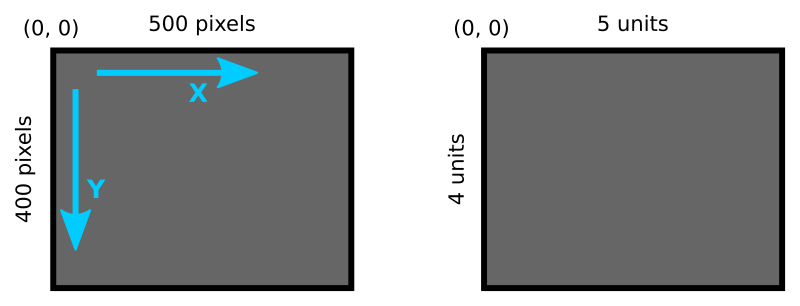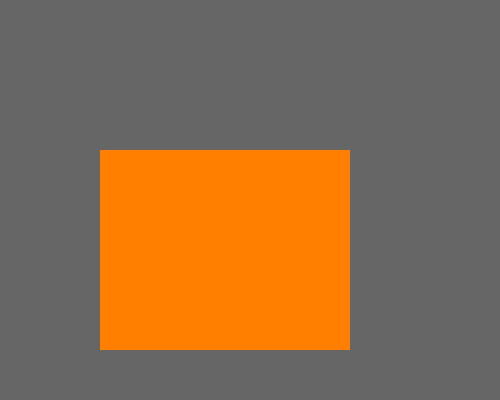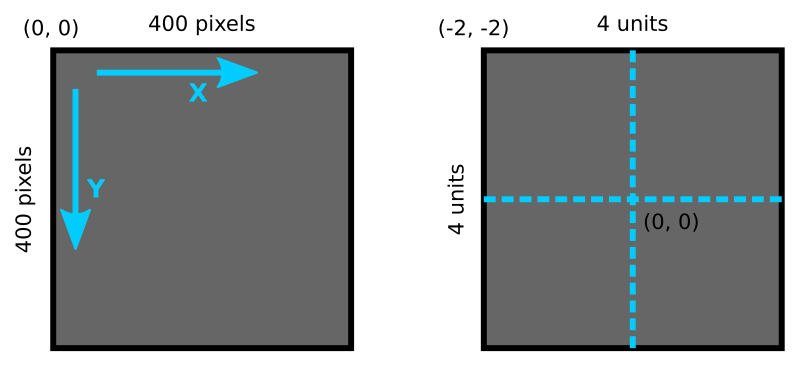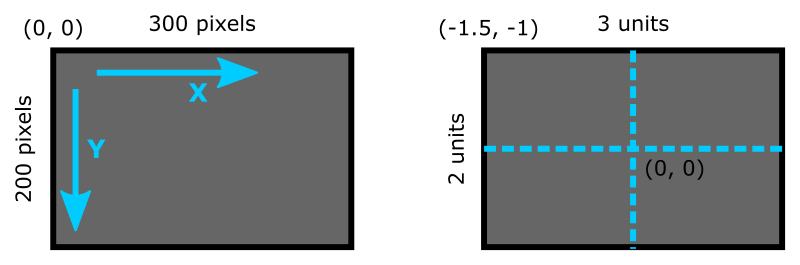# Device and user space in generativepy.drawing

Martin McBride, 2020-08-30
Tags generativepy tutorial device space user space
Categories generativepy generativepy tutorial

In the previous article we used generativepy to create a simple image of a rectangle.

We used pixel coordinates to draw the rectangle. That is to say, we created a rectangle that had a size of 250 by 200 units, and the final image contained a rectangle that was exactly 250 by 200 pixels in size.

That isn't the only option. We can scale our drawing space. For example, we can scale the space by a factor of 100. We can then draw a rectangle taht is 2.5 units by 2 units, and it will appear on the image at 250 by 200 pixels.

This type of scaling has a couple of advantages:

• We can use the most convenient units when we make our drawing. For example, if we wanted to draw a right-angled triangle with sides of 3cm, 4cm and 5cm, we could draw it using lengths of 3, 4 and 5 units. We could then scale it to be whatever pixel size we wanted on the final image.
• If we need to create the same image at different pixel sizes, we can do it very easily. For example we might want a 400 pixel wide image of the triangle for a web page, but a 4000 pixel wide image for a book illustration. We can do this using the same drawing code, just changing the scaling.

This article mainly covers device and user space for vector drawing (ie the drawing and movie modules). There is a section at the end that covers user space for the bitmap module.

## Device and user space.

generatievpy uses the concept of device space and user space to do this scaling (actually it makes direct use of the Pycairo implementation).

Device space is fixed, and always represents the pixel coordinates of the final output image.

User space maps on to device space using a transformation that we can choose in our code.

Whenever we draw anything, we always draw it in user space, and the coordinates get mapped onto device space using the current transform. However, the initial transform is 1:1, so if we never change the transform it appears as if we are drawing in device space.

The transformation between user and device space can include scaling, translation, rotation, mirroring, and shearing in any combination (in fact it can be any affine transformation). You can change the current transform at any time.

## Initialising the user space transform

To make things a bit easier, generativepy allows you to specify the two most commonly used transforms - scaling and translation - in the setup function of the drawing module.

Whether you use this feature or not, you can still make further changes to the transform by using the standard Pycairo functions.

## Scaling user space

In this example, we will see how to scale user space. We will use the previous example of an orange rectangle.

Here is the code:

from generativepy.drawing import make_image, setup
from generativepy.color import Color
from generativepy.geometry import Rectangle, Circle

def draw_rect(ctx, width, height, frame_no, frame_count):
setup(ctx, width, height, width=5, background=Color(0.4))
color = Color(1, 0.5, 0)
Rectangle(ctx).of_corner_size((1, 1.5), 2.5, 2).fill(color)

make_image("rectangle-user.png", draw_rect, 500, 400)


The main difference here is that we have added a width=5 parameter to the setup function.

This tells generativepy to set the user space width to 5 units. Since the device width is 500 pixels, this establishes a user space scaling of 100 - that is, 1 unit in user space maps onto 100 pixels in device space.

The relationship between device and user space is shown here:Here is the image it produces (which is identical to the image created in the previous tutorial, even though the rectangle is expressed in a different user space).The setup function accepts either a width, or height, or both.

• Since the pixel size is 500 by 400, setting width to 5 creates a scale factor of 100, so the user space of 5 by 4 maps onto device space 500 by 400.
• If you prefer you could set the height to 4 instead. This will also create a scale factor of 100, and has exactly the same effect as setting width to 5.
• You can set both width and height. This creates the possibility of having different scale factors in the x and y directions. For example, width=5 and height=8 creates a scale factor of 100 in the x direction but 50 in the y direction. User space of 5 by 8 maps onto device space 500 by 400. This means that objects are "squashed" in the y direction, so for example if you draw a square it will appear as an elongated rectangle. This mode isn't used a often.

## Changing the pixel size of the output image

Suppose we wanted to create a different sized image. Say 2635 by 2108 pixel (totally random size, but in the ratio 5:4). All we need to change is the make_image call:

make_image("rectangle-user.png", draw_rect, 2635, 2108)


This will create an image that has been perfectly scaled up to the new size.

## Scaling and translating user space

In this example we will scale and translate user space.

By default, the origin (0, 0) is always in the top left of the image. That isn't always what you want, and it can be changed like this:

from generativepy.drawing import make_image, setup
from generativepy.color import Color
from generativepy.geometry import Rectangle, Circle

def draw_circle(ctx, width, height, frame_no, frame_count):
setup(ctx, width, height, width=4,
startx=-2, starty=-2, background=Color(0.4))
color = Color("magenta")

make_image("circle-user.png", draw_circle, 400, 400)


This time the pixel size is 400 square, and we have set width=4 so our user space in 4 units square.

But notice that we have also set startx=-2 and starty=-2, which means that the whole user space is shifted by -2 in the x and y directions. The top left of the image is now (-2, -2), which means that the origin (0, 0) is now at the centre of the image, like this:This means that when we draw a circle centred on the origin, it is actually right in the centre of the image:## Other transformations

Here is a quick example of how to use other transformations, such as rotation, using native Pycairo calls.

In this example will will draw the original orange rectangle, but rotated around its top left corner, like this:Here is the code:

from generativepy.drawing import make_image, setup
from generativepy.color import Color
from generativepy.geometry import Rectangle, Circle

def draw_rect_rotated(ctx, width, height, frame_no, frame_count):
setup(ctx, width, height, width=5, background=Color(0.4))

color = Color(1, 0.5, 0)
ctx.save()
ctx.translate(1, 1.5)
ctx.rotate(-0.5)
Rectangle(ctx).of_corner_size((0, 0), 2.5, 2).fill(color)
ctx.restore()

make_image("rotated-rectangle-user.png", draw_rect_rotated, 500, 400)


We have added several Pycairo calls to this code:

• ctx.save() saves the current drawing state. We are about to rotate the coordinate system, we save it here so we can restore it later on.
• ctx.translate(1, 1.5) translates user space so that the origin is at the top left corner of the rectangle.
• ctx.rotate(-0.5) rotates user space by -0.5 radians about the origin. A radian is about 57 degrees, so this is a rotation by almost 30 degrees in the counterclockwise direction. Since we have moved the origin to the top left corner of the rectangle, that is the centre of rotation.

We now draw the rectangle, but we set the corner to be (0, 0) because we previously translation.

• ctx.restore() sets user space back to where it was when save() was called.

Calling save and restore isn't really necessary in this case, because we don't draw anything except the rectangle. But if you were intending to draw more things that you don't want to be rotated, it is very useful to be able to reset things.

## User space with the bitmap module

The bitmap module uses the Python imaging library (PIL) to manipulate bitmap images, rather than using Pycairo to manipulate vector graphics.

PIL works exclusively in pixel coordinates, and does not have any concept of a user space. However, generativepy provides a Scaler class that can perform similar calculations. Here is how it is used:

from generativepy.bitmap import Scaler

scaler = Scaler(300, 200, width=3, height=2, startx=-1.5, starty=-1)

print(scaler.user_to_device(1, .5))   # (250, 150)
print(scaler.device_to_user(20, 50))  # (-1.3, -.5)


The scaler is initialised with a pixel size of 300 by 200, and a user size of 3 by 2 (a scale factor of 100), with a user space offset of (-1.5, -1), like this:The point (1, .5) in user space is converted to device space as ( (1+1.5)100, (0.5+1)100 ), or (250, 150).

The point (20, 50) in device space is converted to user space as ( 20/100 - 1.5, 50/100 -1 ) or (-1.3, -.5).